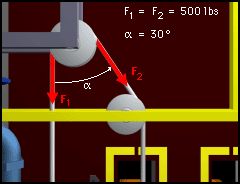Ch 2. Vectors Multimedia Engineering Statics Scalars & Vectors 2-DVectors 3-DVectors DotProducts
 Chapter 1. Basics 2. Vectors 3. Forces 4. Moments 5. Rigid Bodies 6. Structures 7. Centroids/Inertia 8. Internal Loads 9. Friction 10. Work & Energy Appendix Basic Math Units Sections Search eBooks Dynamics Fluids Math Mechanics Statics Thermodynamics Author(s): Kurt Gramoll ©Kurt GramollSTATICS - CASE STUDY IntroductionPulley Force Diagram A pulley assembly is used to move heavy objects around a factory floor. As the assembly moves, the total force on the main pulley changes. At a certain angle, the main pulley fails from the tension in the cable. What is known: The forces on the pulley at failure are as follows: (1) F1 = 500 lb downward (2) F2 = 500 lb, 30° from downward Question What is the total force on the pulley and in what direction does it act? Approach Solve for the rectangular components of the two forces on the pulley. Combine the two forces using vector addition to find the total force exerted on the pulley. Solve for the magnitude and direction of the total force.

Practice Homework and Test problems now available in the 'Eng Statics' mobile app
Includes over 500 problems with complete detailed solutions.
Available now at the Google Play Store and Apple App Store.